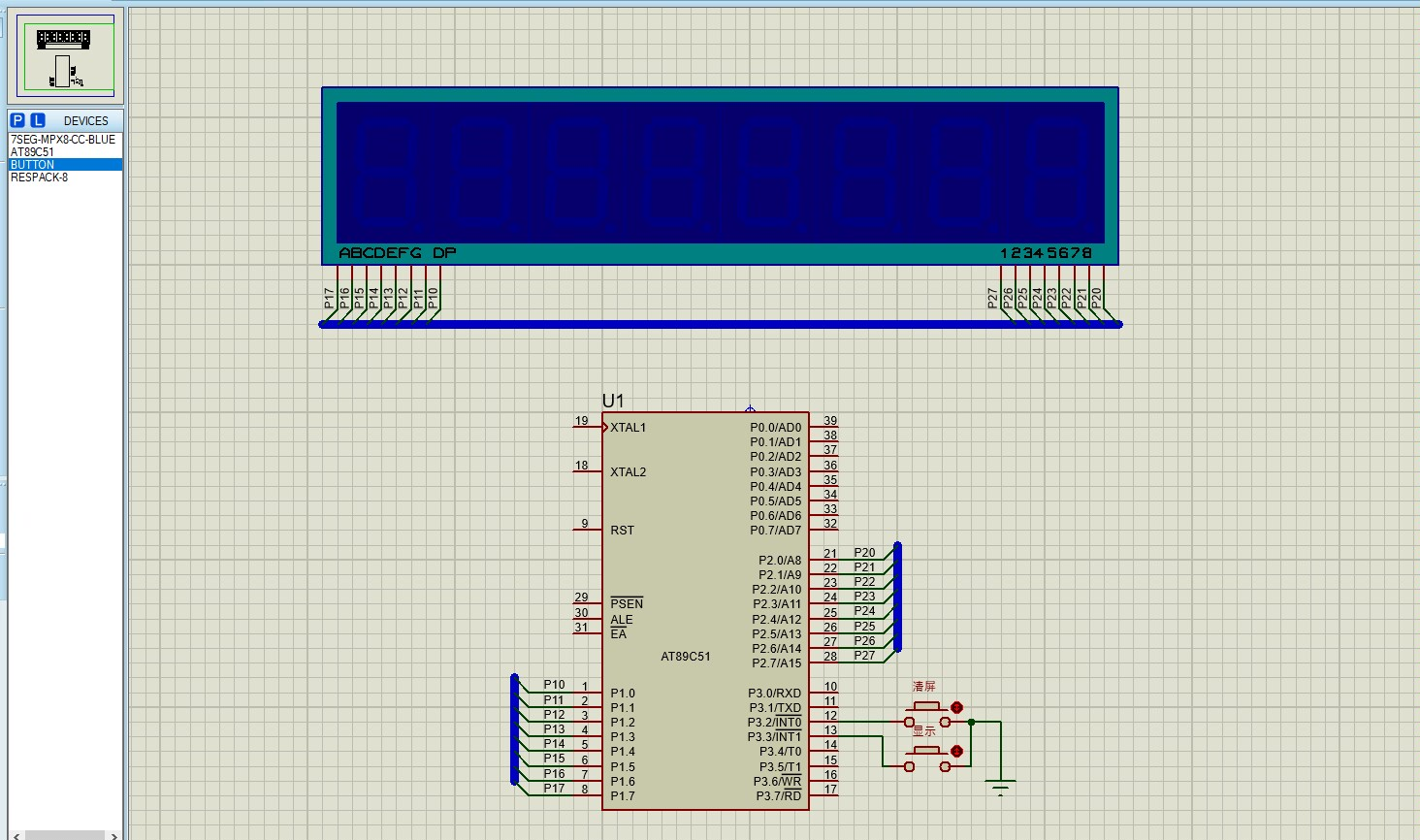“小菜” 2023-05-28 23:17 采纳率: 0%

# 求51单片机8位数码管计时器程序• 写回答

#### 4条回答默认 最新

•瞬间动力 2023-05-28 23:24
关注

花了一个小时写的，希望对你有用！

``````
#include <reg52.h>
#include <intrins.h>

#define uchar unsigned char
#define uint unsigned int

sbit D1 = P2^0;  // 数码管引脚定义
sbit D2 = P2^1;
sbit D3 = P2^2;
sbit D4 = P2^3;
sbit D5 = P2^4;
sbit D6 = P2^5;
sbit D7 = P2^6;
sbit D8 = P2^7;

sbit Key1 = P1^0;  // 按键引脚定义
sbit Key2 = P1^1;

uint ms;    // 毫秒计数器
uint sec;   // 秒计数器
uchar min;  // 分钟计数器
uchar hour; // 小时计数器

void delay(uint i) { // 短暂延时函数
while(i--);
}

void DAC(uint n) { // 将数值DAC输出
D1 = (n & 0x01);
D2 = (n & 0x02) >> 1;
D3 = (n & 0x04) >> 2;
D4 = (n & 0x08) >> 3;
D5 = (n & 0x10) >> 4;
D6 = (n & 0x20) >> 5;
D7 = (n & 0x40) >> 6;
D8 = (n & 0x80) >> 7;
}

void display() { // 显示计时器数值
DAC(hour / 10); // 输出小时的十位
delay(50); // 短暂延时
DAC(hour % 10); // 输出小时的个位
delay(50);
DAC(min / 10); // 输出分钟的十位
delay(50);
DAC(min % 10); // 输出分钟的个位
delay(50);
DAC(sec / 10); // 输出秒的十位
delay(50);
DAC(sec % 10); // 输出秒的个位
delay(50);
}

void initTimer() { // 初始化定时器
TMOD |= 0x01; // 设置定时器0为16位模式，使用定时器0的模式1
TH0 = 0xFC; // 计时1ms，从0xFC开始（65536-1000）计时
TL0 = 0x18;
ET0 = 1; // 允许定时器0中断
TR0 = 1; // 启动定时器0
EA = 1; // 打开总中断开关
}

void main() {
initTimer(); // 初始化定时器
while(1) {
if (Key1 == 0) { // 如果按下了按键1，复位计时器
ms = sec = min = hour = 0;
display(); // 显示0
}
if (Key2 == 0) { // 如果按下了按键2，开始计时器
while(1) {
display(); // 显示计时器数值
if (ms >= 1000) {
ms = 0;
sec++;
if (sec >= 60) {
sec = 0;
min++;
if (min >= 60) {
min = 0;
hour++;
if (hour >= 24) {
hour = 0;
}
}
}
}
}
}
}
}

void timer0() interrupt 1 { // 定时器0中断
TH0 = 0xFC;
TL0 = 0x18;
ms++; // 每次中断毫秒计数器加1
}
``````
评论

#### 悬赏问题

• ¥15 vue引入sdk后的回调问题
• ¥15 求一个智能家居控制的代码
• ¥15 ad软件 pcb布线pcb规则约束编辑器where the object matpcb布线pcb规则约束编辑器where the object matchs怎么没有+15v只有no net
• ¥15 虚拟机vmnet8 nat模式可以ping通主机，主机也能ping通虚拟机，但是vmnet8一直未识别怎么解决，其次诊断结果就是默认网关不可用
• ¥20 求各位能用我能理解的话回答超级简单的一些问题
• ¥15 yolov5双目识别输出坐标代码报错
• ¥15 这个代码有什么语法错误
• ¥15 给予STM32按键中断与串口通信
• ¥15 使用QT实现can通信
• ¥15 关于sp验证的一些东西，求告知如何解决，